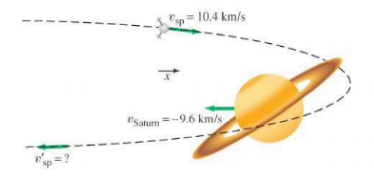×
Get Full Access to Physics: Principles With Applications - 6 Edition - Chapter 7 - Problem 81gp
Get Full Access to Physics: Principles With Applications - 6 Edition - Chapter 7 - Problem 81gp

×

# The gravitational slingshot effect. Figure 7-51 shows theISBN: 9780130606204 3

## Solution for problem 81GP Chapter 7

Physics: Principles with Applications | 6th Edition

• Textbook Solutions
• 2901 Step-by-step solutions solved by professors and subject experts
• Get 24/7 help from StudySoup virtual teaching assistantsPhysics: Principles with Applications | 6th Edition

4 5 1 260 Reviews
14
0
Problem 81GP

The gravitational slingshot effect. Figureshows the planet Saturn moving in the negativedirection at its orbital speed (with respect to the Sun) of $$9.6 \mathrm{~km} / \mathrm{s}$$. The mass of Saturn is $$5.69 \times 10^{26} \mathrm{~kg}$$. A spacecraft with massapproaches Saturn. When far from Saturn, it moves in thedirection at $$10.4 \mathrm{~km} / \mathrm{s}$$. The gravitational attraction of Saturn (a conservative force) acting on the spacecraft causes it to swing around the planet (orbit shown as dashed line) and head off in the opposite direction. Estimate the final speed of the spacecraft after it is far enough away to be considered free of Saturn's gravitational pull.FIGURE 7-47 Problem 81

Equation Transcription:Text Transcription:

9.6 km/s

5.69 x 10^26 kg

10.4 km/s

Step-by-Step Solution:

Solution 81GP:

We have to determine the final speed with which spacecraft was moving, as its direction of motion was reversed due to gravitational attraction between the spacecraft and Saturn.

Step 1 of 5

Concept:

The interaction between the Saturn and the spacecraft is elastic, because the force of gravity is conservative. Thus kinetic energy is conserved in this interaction.

Relative velocity of body A with respect to body B having velocityrespectively, is given as,Step 2 of 5

Step 3 of 5

## Discover and learn what students are asking

Calculus: Early Transcendental Functions : First-Order Linear Differential Equations
?In Exercises 5-14, solve the first-order linear differential equation. $$\frac{d y}{d x}+\left(\frac{1}{x}\right) y=6 x+2$$

Calculus: Early Transcendental Functions : Partial Derivatives
?In Exercises 7 - 38, find both first partial derivatives. $$z=\ln \frac{x}{y}$$

Statistics: Informed Decisions Using Data : Testing the Significance of the Least-Squares Regression Model
?The output shown was obtained from Minitab. (a) The least-squares regression equation is yn = 1.3962x + 12.396. What is the predict

Statistics: Informed Decisions Using Data : Inference about Measures of Central Tendency
?Write a paragraph that describes the logic of the test statistic in a right-tailed sign test.

Unlock Textbook Solution